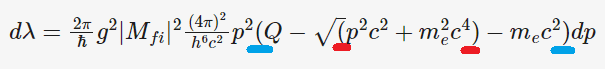# Fermi Golden Rule Derivation

• I
• James_1978

#### James_1978

Dear Forum,

I have a question about the derivation of the Fermi golden rule in Kenneth Krane's Introduction to Nuclear Physics. I understand everything up to equation 9.20. However, it is unclear how he goes directly to equation 9.21. Here is equation 9.20,

## d\lambda = \frac{2\pi}{\hbar}g^{2} |M_{fi}|^{2} \frac{(4\pi)^{2}}{h^{6}c^{2}} p^{2} (Q - \sqrt(p^{2}c^{2} + m_{e}^{2}c^{4}) -m_{e}c^{2})dp##

Which is then followed by equation 9.21,

## N(p)dp = Cp^{2}q^{2}dp ##

I under stand that C has the constants not related to p. My question is how did he go from ##d\lambda## to ##N(p)dp##. This says that ##d\lambda = N(p)dp## which is unclear to me. Can someone explain that part to me.

Thank you.

9.20 doesn't look right. LHS is a differential. RHS has two terms: the first is not a differential, second is. Can't be.

•PeroK
RHS has two terms: the first is not a differential
Terms?

There is a dp though

dλ=2πℏg2|Mfi|2(4π)2h6c2p2(Q−(p2c2+me2c4)−mec2)dp
I think the expression you have in brackets here should be squared (what you wrote equals ##q## by 9.22, but in 9.20 it is ##q^2##).

I am not sure there is much to understand here,

##d\lambda = N(p) dp##

simply defines ##N(p)## as far as I can tell. The only part of

##N(p) = C p^2 q^2##

that might be non-trivial is that indeed all other factors (in particular ##|M_{fi}|##) are independent of ##p^2##. However the text states this is an assumption at this point. Could you clarify what is unclear?

Terms?

There is a dp though
RHS is the sum of two terms. The second term has dp. The first term has no differential - it needs one.

I think you are misreading the brackets (one is opened before the ##Q##, and the corresponding closed one is right before the ##dp##...)

•malawi_glenn
RHS is the sum of two terms. The second term has dp. The first term has no differential - it needs one.
No RHS has three terms.
Note that the OP does not know LaTeX and has this written sqrt wrong##d\lambda = \dfrac{2\pi}{\hbar} g^2 |M_{fi}|^2 \dfrac{(4\pi)^2}{h^6c^2}p^2 (Q - \sqrt{p^2c^2+m_e^2c^4} -m_ec^2) dp##

Last edited:
No RHS has three terms.
Note that the OP does not know LaTeX and has this written sqrt wrong
View attachment 324103
•